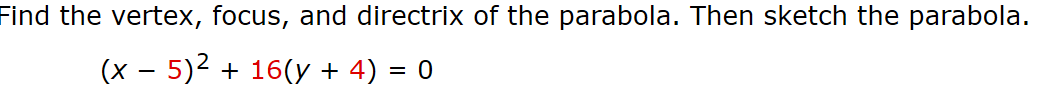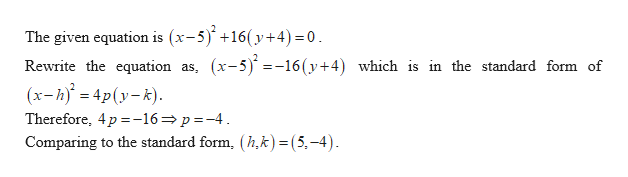# Find the vertex, focus, and directrix of the parabola. Then sketch the parabola.(x 5)216(y4) 0

Question
1 views

I dont understand if this is a completing a square problem and if so how i would go about solving it.help_outlineImage TranscriptioncloseFind the vertex, focus, and directrix of the parabola. Then sketch the parabola. (x 5)2 16(y4) 0 fullscreen
check_circle

Step 1help_outlineImage Transcriptionclose(x-5)+16(y+4)= 0 The given equation is (x-5 Rewrite the equation as, (x-5) =-16(y+4) which is in the standard form of (x-h 4py-k) Therefore, 4p -16p-4 Comparing to the standard form, (h,k) = (5,-4) fullscreen
Step 2

### Want to see the full answer?

See Solution

#### Want to see this answer and more?

Solutions are written by subject experts who are available 24/7. Questions are typically answered within 1 hour.*

See Solution
*Response times may vary by subject and question.
Tagged in

### Calculus